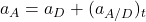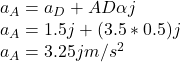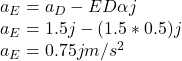A 5-m steel beam is lowered by means of two cables unwinding at the same speed from overhead cranes. As the beam approaches the ground, the

Question

A 5-m steel beam is lowered by means of two cables unwinding at the same speed from overhead cranes. As the beam approaches the ground, the crane operators apply brakes to slow the unwinding motion.

At the instant considered, the deceleration of the cable attached at B is 2.5 m/s2 , while that of the cable attached at D is 1.5 m/s2 .

Determine

(a) the angular acceleration of the beam,
(b) the acceleration of points A and E.

in progress 0
6 months 2021-07-19T22:04:46+00:00 1 Answers 12 views 0

a) The angular acceleration of the beam is 0.5 rad/s²CW (direction clockwise due the tangential acceleration is positive)

b) The acceleration of point A is 3.25 m/s²

The acceleration of point E is 0.75 m/s²

Explanation:

a) The relative acceleration of B with respect to D is equal: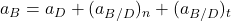Where

aB = absolute acceleration of point B = 2.5 j (m/s²)

aD = absolute acceleration of point D = 1.5 j (m/s²)

(aB/D)n = relative acceleration of point B respect to D (normal direction BD) = 0, no angular velocity of the beam

(aB/D)t = relative acceleration of point B respect to D (tangential direction BD)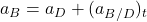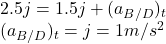We have that

(aB/D)t = BDα

Where α = acceleration of the beam

BDα = 1 m/s²

Where

BD = 2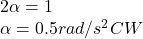b) The acceleration of point A is: